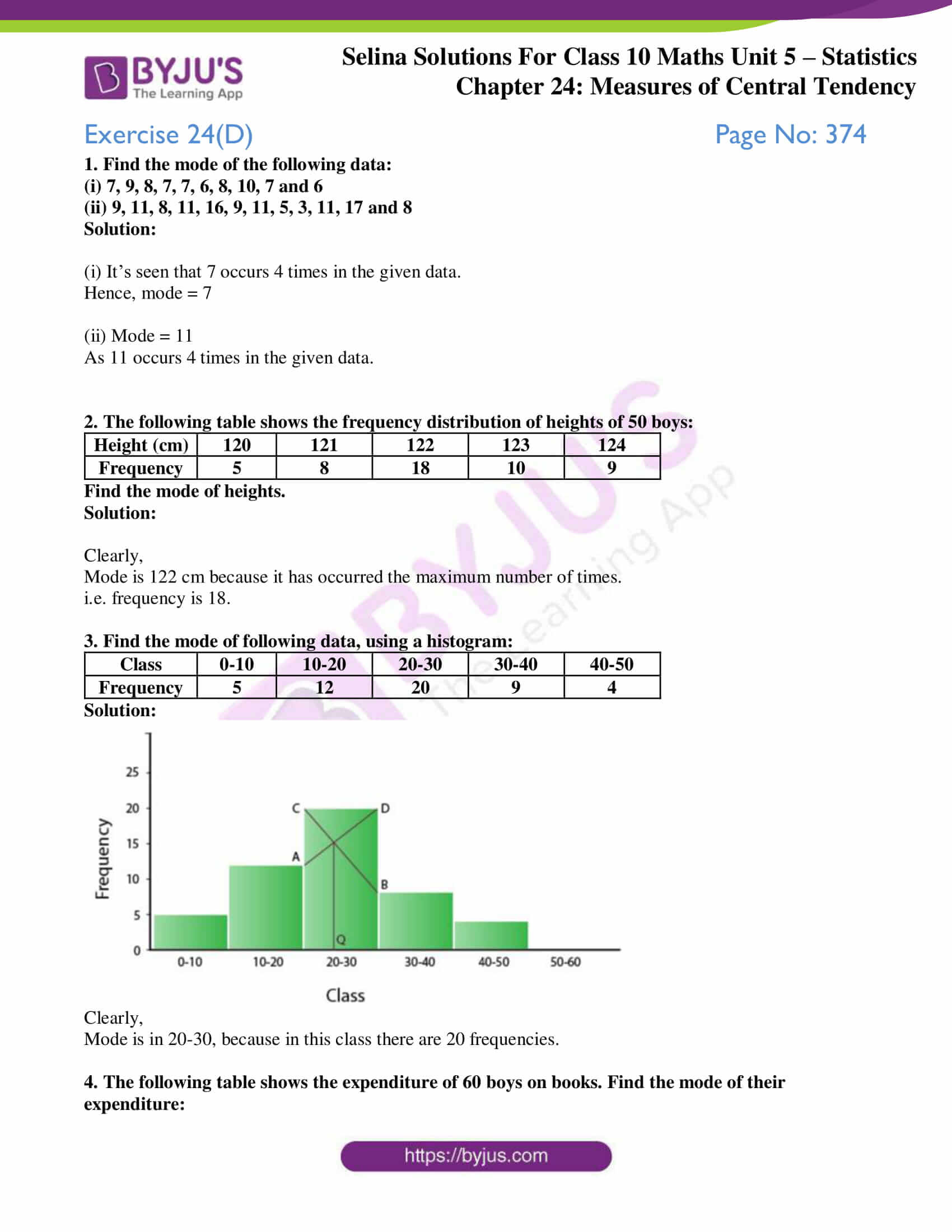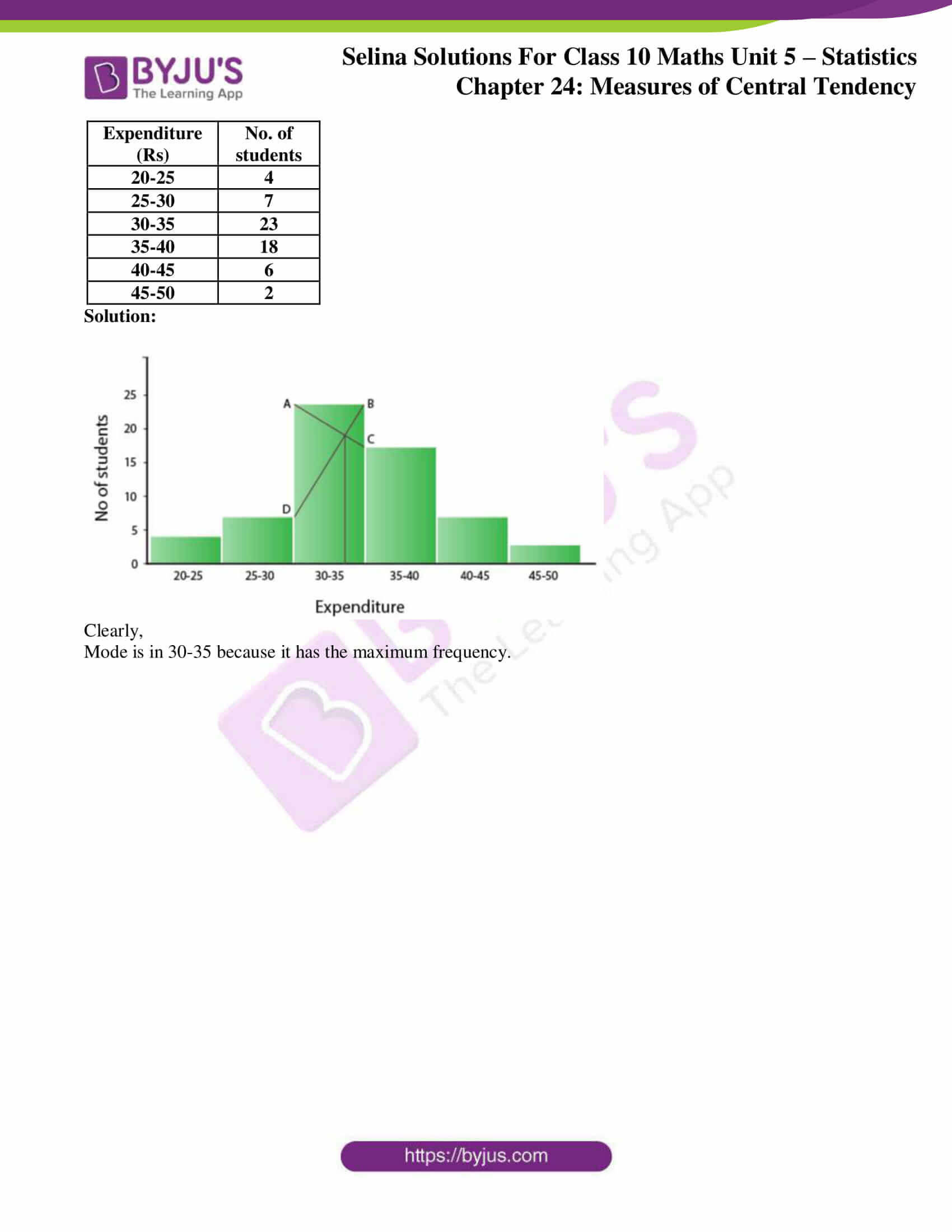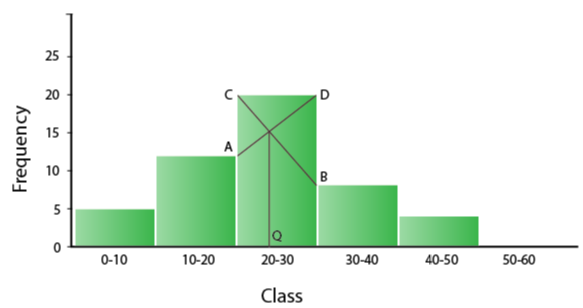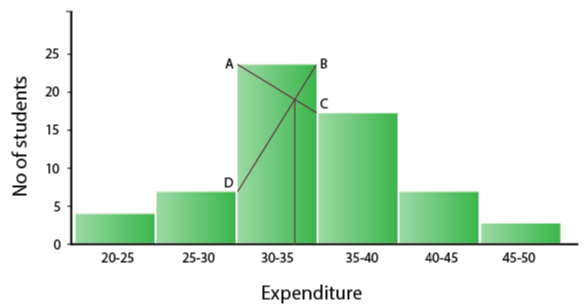# Selina Solutions Concise Maths Class 10 Chapter 24 Measures of Central Tendency Exercise 24(D)

Finding the mode of the given raw, tabulated and grouped data are the major insights gained from this exercise.The Selina Solutions for Class 10 Maths is one valuable resource for students to clear doubts when solving problems of this chapter and others. All the solutions are as per the latest ICSE patterns. The solutions of this exercise can be accessed in the Concise Selina Solutions for Class 10 Maths Chapter 24 Measures of Central Tendency Exercise 24(D) PDF, provided in the link enclosed below.

## Selina Solutions Concise Maths Class 10 Chapter 24 Measures of Central Tendency Exercise 24(D) Download PDF### Access Selina Solutions Concise Maths Class 10 Chapter 24 Measures of Central Tendency Exercise 24(D)

1. Find the mode of the following data:

(i) 7, 9, 8, 7, 7, 6, 8, 10, 7 and 6

(ii) 9, 11, 8, 11, 16, 9, 11, 5, 3, 11, 17 and 8

Solution:

(i) It’s seen that 7 occurs 4 times in the given data.

Hence, mode = 7

(ii) Mode = 11

As 11 occurs 4 times in the given data.

2. The following table shows the frequency distribution of heights of 50 boys:

 Height (cm) 120 121 122 123 124 Frequency 5 8 18 10 9

Find the mode of heights.

Solution:

Clearly,

Mode is 122 cm because it has occurred the maximum number of times.

i.e. frequency is 18.

3. Find the mode of following data, using a histogram:

 Class 0-10 10-20 20-30 30-40 40-50 Frequency 5 12 20 9 4

Solution:Clearly,

Mode is in 20-30, because in this class there are 20 frequencies.

4. The following table shows the expenditure of 60 boys on books. Find the mode of their expenditure:

 Expenditure (Rs) No. of students 20-25 4 25-30 7 30-35 23 35-40 18 40-45 6 45-50 2

Solution:Clearly,

Mode is in 30-35 because it has the maximum frequency.